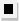Chapter 13.CR, Problem 71E### Algebra and Trigonometry (MindTap ...

4th Edition
James Stewart + 2 others
ISBN: 9781305071742

#### Solutions

Chapter
Section### Algebra and Trigonometry (MindTap ...

4th Edition
James Stewart + 2 others
ISBN: 9781305071742
Textbook Problem

#7 0 - 7 2 Proof by Induction Use mathematical induction to show that the given statement is true.The Fibonacci number F 4 n is divisible by 3 for all natural numbers n .

To determine

To prove:

The Fibonacci number F4n is divisible by 3 using mathematical induction.

Explanation

Given:

The Fibonacci number is given as,

F4n

Approach:

Prove basic step for n=1. Assume the statement is true for some natural number n1 and show that the statement is true for n+1.

Calculation:

Denote the statement “The Fibonacci number F4n is divisible by 3” by P(n).

Step 1:

Since,

F1=1F2=1F3=F1+F2=1+1

F4=F3+F2=2+1=3

For, n=1

This proves Step 1.

Step 2:

Assume P(n) is true for some n1. This is called the induction hypothesis.

Show that P(n+1) is true.

Since induction hypothesis is true hence F4n is divisible by 3 and can be written as F4n=3k for some integer k

### Still sussing out bartleby?

Check out a sample textbook solution.

See a sample solution

#### The Solution to Your Study Problems

Bartleby provides explanations to thousands of textbook problems written by our experts, many with advanced degrees!

Get Started